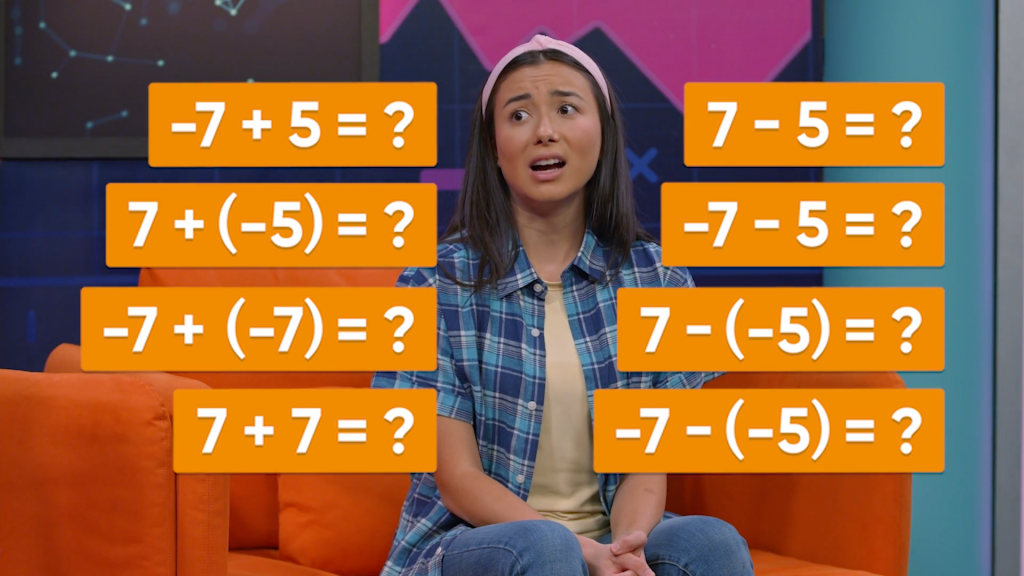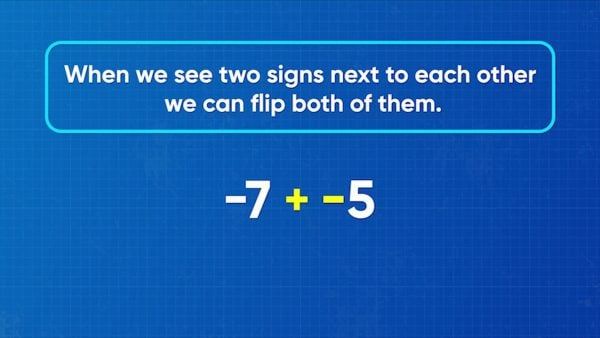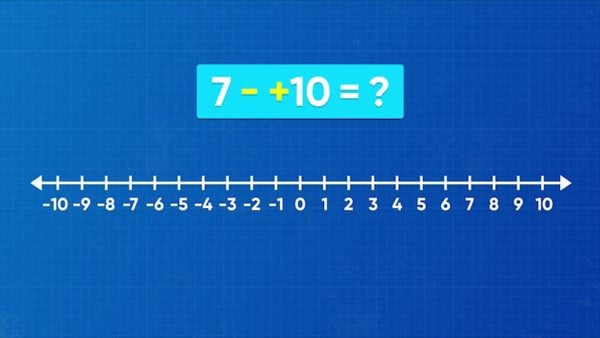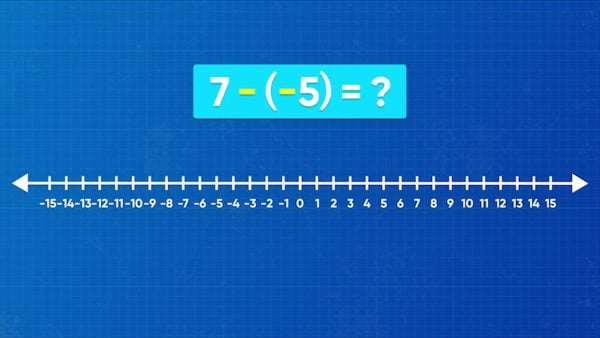Add & Subtract with Negative Numbers Math Video for Kids - Grades 6-8
1%
It was processed successfully!HOW DO YOU ADD & SUBTRACT NEGATIVE NUMBERS?

You have seen how to use a number line to represent negative numbers. In this lesson, you learn how to use the number line to show addition and subtraction of negative numbers.

To better understand adding & subtracting negative numbers…

HOW DO YOU ADD & SUBTRACT NEGATIVE NUMBERS?. You have seen how to use a number line to represent negative numbers. In this lesson, you learn how to use the number line to show addition and subtraction of negative numbers. To better understand adding & subtracting negative numbers…

## LET’S BREAK IT DOWN!There is one rule we can use when we add and subtract integers: When we see 2 signs next to each other, we can flip both of them to make a simpler problem with the same answer. For example, –7 + (–5) is the same as –7 – (+5) which is the same as –7 – 5. –7 – 5 = –12. This rule takes problems where you see 2 signs next to each other and lets you show 1 operation instead. When using this rule, the remaining one operation tells you which way to move on the number line: to add, move to the right; to subtract, move to the left. Try this one yourself. Use the rule to find 5 + (–4).

You can use a quick rule to help you add and subtract integers. There is one rule we can use when we add and subtract integers: When we see 2 signs next to each other, we can flip both of them to make a simpler problem with the same answer. For example, –7 + (–5) is the same as –7 – (+5) which is the same as –7 – 5. –7 – 5 = –12. This rule takes problems where you see 2 signs next to each other and lets you show 1 operation instead. When using this rule, the remaining one operation tells you which way to move on the number line: to add, move to the right; to subtract, move to the left. Try this one yourself. Use the rule to find 5 + (–4).

### Use the rule to add a negative number.Here’s another example: 7 + (–10). An equivalent way to write this problem is 7 – (+10), which is the same as 7 – 10. On a number line, you can start at 7 and then subtract 10 by moving 10 units to the left. The number you land on is –3. 7 + (–10) = –3. When subtracting in problems like these it’s important to think about which side of zero you end up on. If you end up to the left of 0, the answer is negative. If you end up to the right of zero, it’s positive. Try this one yourself. Find 8 + (–9).

Use the rule to add a negative number. Here’s another example: 7 + (–10). An equivalent way to write this problem is 7 – (+10), which is the same as 7 – 10. On a number line, you can start at 7 and then subtract 10 by moving 10 units to the left. The number you land on is –3. 7 + (–10) = –3. When subtracting in problems like these it’s important to think about which side of zero you end up on. If you end up to the left of 0, the answer is negative. If you end up to the right of zero, it’s positive. Try this one yourself. Find 8 + (–9).

### Use the rule to subtract a negative number.Suppose you need to solve the problem 7 – (–5). In this problem, there are two negative signs next to each other. When you use the rule, you can write this problem as 7 + (+5) or 7 + 5. Then the problem involves adding two positive numbers: 7 + 5 = 12. On a number line, start 7 units to the right of 0 and then move 5 units to the right. Here’s another problem: – 7 – (–5). Flip the two negative signs that are next to each other to get –7 + (+5), which is the same as –7 + 5. Move to –7 on the number line and then move 5 units in the positive direction. Land on –2. Always remember, the left and right rules only work when the second number is positive. Try this one yourself. Find 3 – (–4).

Use the rule to subtract a negative number. Suppose you need to solve the problem 7 – (–5). In this problem, there are two negative signs next to each other. When you use the rule, you can write this problem as 7 + (+5) or 7 + 5. Then the problem involves adding two positive numbers: 7 + 5 = 12. On a number line, start 7 units to the right of 0 and then move 5 units to the right. Here’s another problem: – 7 – (–5). Flip the two negative signs that are next to each other to get –7 + (+5), which is the same as –7 + 5. Move to –7 on the number line and then move 5 units in the positive direction. Land on –2. Always remember, the left and right rules only work when the second number is positive. Try this one yourself. Find 3 – (–4).

### Add and subtract integers to solve real-world problems.You are a scuba diver starting at sea level, which we call zero. You dive down 8 meters, and then another 4 meters. How deep are you? You can represent the problem as –8 – 4. On the number line, that would be 8 to the left and then another 4 to the left. You land on –12. You are at a depth of –12 meters. Next, you are going hiking. You start 8 meters above sea level and end the hike in a valley, at 2 meters below sea level. What is the difference in elevation between the start and end of the hike? You need to find 8 – (–2). Use the rule to find that this is the same as 8 + 2, which is 10. Your elevation changed by 10 meters. Try this one yourself. You are going hiking. You start 4 meters below sea level and end the hike at 8 meters above sea level. What is the difference in elevation between the start and end of the hike?

Add and subtract integers to solve real-world problems. You are a scuba diver starting at sea level, which we call zero. You dive down 8 meters, and then another 4 meters. How deep are you? You can represent the problem as –8 – 4. On the number line, that would be 8 to the left and then another 4 to the left. You land on –12. You are at a depth of –12 meters. Next, you are going hiking. You start 8 meters above sea level and end the hike in a valley, at 2 meters below sea level. What is the difference in elevation between the start and end of the hike? You need to find 8 – (–2). Use the rule to find that this is the same as 8 + 2, which is 10. Your elevation changed by 10 meters. Try this one yourself. You are going hiking. You start 4 meters below sea level and end the hike at 8 meters above sea level. What is the difference in elevation between the start and end of the hike?

## ADD & SUBTRACT WITH NEGATIVE NUMBERS VOCABULARY

Negative numbers
The numbers to the left of 0 on a number line.
Positive numbers
The numbers to the right of 0 on a number line.
Number line
A line on which numbers are marked in order and at equal intervals.
Integers
Zero, all the whole numbers, and their opposites.
Sign
Identifies a number as positive or negative.
Operation
The four operations are addition, subtraction, multiplication, and division.

## ADD & SUBTRACT WITH NEGATIVE NUMBERS DISCUSSION QUESTIONS

### Give students the problem 5 + 7 and have them show the solution on the number line.

A number line shows that the solution to 5 + 7 is 12. Start at 5 and jump 7 units to the right, to 12.

### Give students the problem –5 + (–8) and have them show the solution on the number line.

A number line shows that the solution to –5 + (–8) is –13. Start at –5 and jump 8 units to the left, to –13.

### Give students the problem +3 – 7 and have them show the solution on the number line.

A number line shows that the solution to +3 – 7 is –4. Start at 3 and jump 7 units to the left, to –4.

### Give students the problem –4 + (–3) and have them show the solution on the number line.

A number line shows that the solution to –4 + (–3) is –7. Start at –4 and jump 3 units to the left, to –7.

### Give students the problem 4 – (–5) and have them show the solution on the number line.

A number line shows that the solution to 4 – (–5) is 9. Start at 4 and jump 5 units to the right, to 9.
X

## Success

We’ve sent you an email with instructions how to reset your password.
Ok